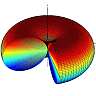## The Infinitesimal Dipole - Radiation Pattern, Directivity and all thatIn this problem we first investigate the radiation properties of a very short and thin filament of current. Although this filament of current as an antenna is not physically realizable, as a mathematical model it will help us to understand the general behavior of antennas. Here we also introduce some of the fundamental antenna parameters.

## Problem Statement

A very thin and short antenna which works at frequency $$\omega$$ has the the following current distribution.

$\mathbf{J}\left(\mathbf{r},\omega\right)=\mathbf{J_0} \, \delta (\mathbf{r})=J_0 \, \delta (\mathbf{r}) \mathbf{\hat{z}}$

where $$J_0$$ is the amplitude of the current distribution, $$\delta (\mathbf{r})$$ is delta function, and $$\mathbf{\hat{z}}$$ is the unit vector in z direction of the Cartesian coordinate . Assume that the antenna (current distribution) is placed in a homogeneous environment with permitivity and permeability of $$\varepsilon$$ and $$\mu$$.

1. Find the generated electric and magnetic fields $$\big(\mathbf{E}\left(\mathbf{r},\omega\right), \mathbf{H}\left(\mathbf{r},\omega\right)\big)$$ by the antenna.

2. Sketch the far filed radiation pattern of the antenna in transverse and vertical planes (e.g. x-y and x-z planes).

3. Calculate the half power beamwidth (HW) of the antenna in x-z plane and its directivity.